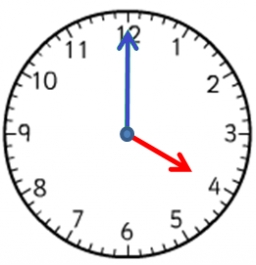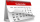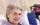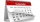# Greg and Bill

Greg is 18 years old. He is 6 less than 4 times Bill's age. How old is Bill?

b =  6

### Step-by-step explanation:Did you find an error or inaccuracy? Feel free to write us. Thank you!Tips to related online calculators
Do you want to convert time units like minutes to seconds?

## Related math problems and questions:

• Age questionJano is three times older than Zdeno. Zdeno is 12 years younger than Janko. How old is Jano, and how old is Zdeno?
• Maya isMaya is 14 years old. Her brother Jorge is 3 years more than half her age. Which of the following is the correct expression for Jorge's age?
• FamilyMom is 39 years old. Karl is eight years and Paul 4 years. For many years mother would old just like two children together?
• TimeageSeven times my age is 8, less than the largest two-digit number. How old am I?
• Bed timeTiffany was 5 years old; her week night bedtime grew by ¼ hour each year. If, at age 18, her curfew time is 11pm, what was her bed time when she was 5 years old?
• Age problemsA) Alex is 3 times as old as he was 2 years ago. How old is he now? b) Casey was twice as old as his sister 3 years ago. Now he is 5 years older than his sister. How old is Casey? c) Jessica is 4 years younger than Jennifer now. In 10 years, Jessica will
• SuzanSusan's age will be after 12 years four times as much as twelve years ago. How old is Susan now?
• How oldThe student who asked how many years he answered: "After 10 years I will be twice as old than as I was four years ago. How old is student?
• Lee isLee is 8 years more than twice Park's age, 4 years ago, Lee was three times as old. How old was Lee 4 years ago?
• Two dogsIzzy's dog is 10 1/2 years old. Paige's dog is 18 months old. How many years older is Izzy's dog?
• TranslateTranslate the following mathematical statement into an algebraic expression or equation. 1. Nine less that the quotient of a number and 3 2. The ratio of 3 and the sum of 4 and an unknown number 3. Eighty is the product of 4 and g 4. The sum of x and 18 i
• Expressions with variableThis is algebra. Let n represent an unknown number and write the following expressions: 1. 4 times the sum of 7 and the number x 2. 4 times 7 plus the number x 3. 7 less than the product of 4 and the number x 4. 7 times the quantity 4 more than the number
• Three children3 children eat 8 chocolates in 6 days. How many chocolates 6 children eat in 18 days?
• How many 15How many six-eights are there in 18? Which of the following would you choose to solve this question? 18 x 6/8 or 18 : 6/8
• Grandfather and grandmotherThe old mother is 5 years younger than the old father. Together they are 153 years old. How many years has each of them?
• Family ageKubo is 14 years old and has a one-year-old sister Nina and four-year-old brother Sam. How many years will Nina and Sam be as old as Kubo?
• Four paversFour pavers would pave the square in 18 days. How many pavers do you need to add to done work in 12 days?